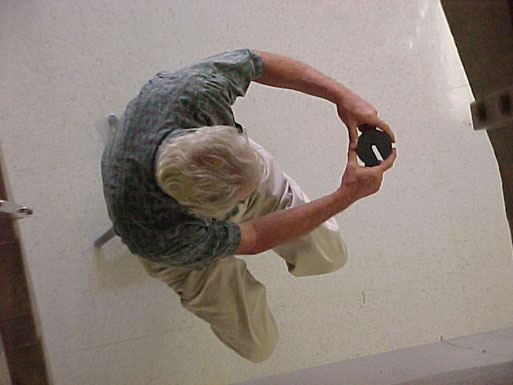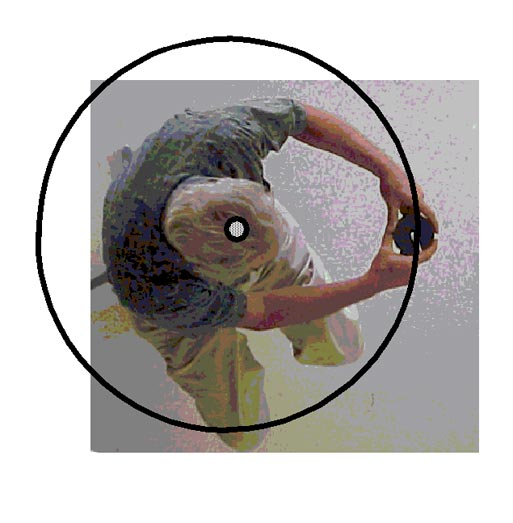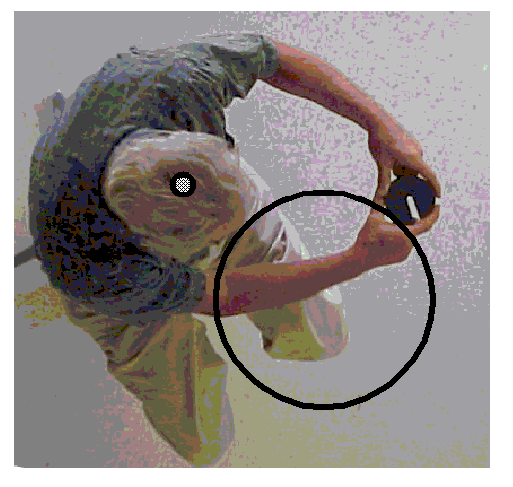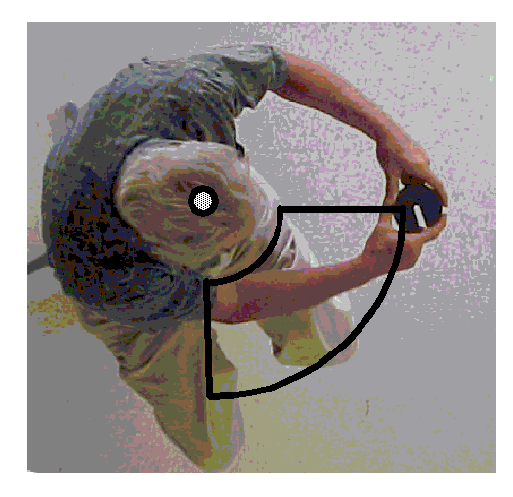# Experiment of The Month

## Free Space Reorientation

The photo below illustrates a lecture demonstration of a surprising fact: If you are lost in space, with nothing to touch, you can still change your orientation. If you are facing away from earth, you can change so that you are able to see the earth.To make the discussion concrete, Dr. Miziumski holds a weight at arm's length as he sits on a stool with a rotating seat. The seat rotates about a vertical axis. We will assume that angular momentum is conserved, and is zero, for the system consisting of Dr. Miziumski, the stool, and the weight.In the picture above, the dot on top of Dr. Miziumski's head represents the center of rotation of the chair-professor-weight system. If he were able to rotate the weight clockwise, in the circle shown, the rest of the system would rotate counterclockwise, keeping the system angular momentum constant at zero. A smaller version of such a circle can be created, by holding the arms above the head.

There is no need for the weight to move in such a circle, however. The weight can be moved clockwise in the smaller circle shown below. The angular momentum of the system would still remain zero, and the stool would have to rotate counterclockwise to make that happen.For a blow-by-blow discussion, it is easier to picture the motion of the weight as shown below.The sense of the motion of the weight is still clockwise, but the path now consists of two circular arcs plus two straight lines. The arcs are centered on the axis of rotation. The straight lines are radial; if extended, each would pass through the center of rotation. In the picture, the moment of inertia of the weight is its mass multiplied by the *square of* the radial distance to the axis of rotation.

Along both arcs, the weight moves through an angle of the same size, but opposite direction. It will help to imagine that the rate of motion (as seen by an observer in looking down on the experiment) has the same magnitude for both arcs, so that the time spent moving along the large arc is the same as the time spent moving along the small arc. The magnitude of the angular velocity is the same for both arc motions.

When the weight is moved clockwise along the larger arc, its angular momentum is the product of the moment of inertia and its angular velocity. The angular momentum of the professor-plus-chair must have the same magnitude, but opposite sense. The professor-plus-chair rotates with a (smaller) counterclockwise angular velocity. During the time that the weight moves on the large arc, the professor rotates through a (smaller) angle, counterclockwise.

Next, the weight is moved inward along the radial line. This radial motion produces no motion of the professor-plus-chair.

Next, the weight moves counterclockwise along the small arc, through the same angle as the large arc, but with opposite sense. The moment of inertia of the weight is now *smaller*, and (for the same angular velocity) so is its angular momentum. To keep angular momentum constant, the professor-plus-chair rotates clockwise with a *smaller* angular velocity. (Note; the professor-plus-chair moment of inertia is take to be unchanged.) This is true even though the angular velocity of the weight has the same magnitude as before.

Since it moves at the same angular speed, for the same time, the weight moves counterclockwise through an angle of the same size as before. However (because his angular velocity is less) the professor-plus-chair moves clockwise through a *smaller* angle than before. The second angular motion of the weight "undoes" the first angular motion of the weight. However, the second angular motion of the professor is too small to "undo" the first angular motion of the professor.

Motion out along the radial line completes a cycle.

During the cycle, the professor-plus-chair has rotated in the counterclockwise direction, by an angle equal to the difference between the counterclockwise and clockwise rotations.

## Experiment Of The Month

View past labs experiments.